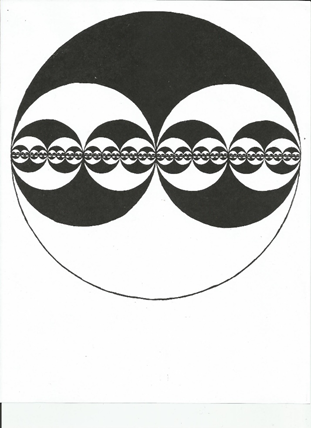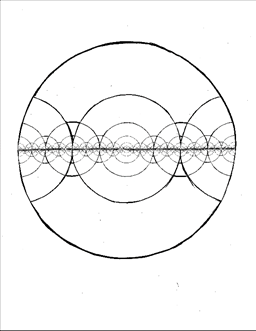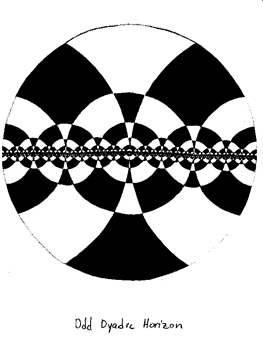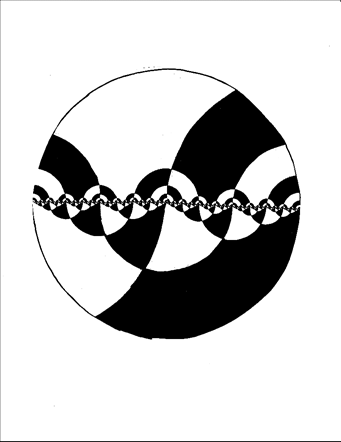## Monday, May 17, 2021

### Teacher’s Riddle

Teacher’s Riddle

What is the difference between a plagiarist and a disciple?

A plagiarist impoverishes the teacher; a disciple impoverishes the teachings.

Therefore a good teacher loves a disciple, but respects a plagiarist.

## Friday, May 14, 2021

### Logistic Fractal

Consider this iterative system:

A = 1/A + B

B = 1/B + C

C = 1/C + 1/A

If we use the logistic interpretation:  0  =  True,  Infinity = False,  + = 'and',  1/x = 'not', then this translates into this paradox system:

A   =   B and not A

B   =   C and not B

C   =   not A and not C

I have experimented with this system, and find that whatever initial values I input for ABC, it quickly converges to a period-2 oscillation. But not the _same_ oscillation for different initial values!

My question is: what does the set of period-2 wobbles look like in 3d space? Can you do this: Program a computer to take a large number of random points, iterate all to a wobble, plot all the resulting point pairs, and display it, as seen along the three axes, as gifs. Or perhaps some stereograms?

Iterated twice, the system is:

A    =   (A {+} b) + (b + C)

b     =   (b + C) {+} (C {+} A)

C    =   (C {+} A) + (A {+} b)

where b  =  1/B, and {+} is the reciprocal addition operator 1/((1/x)+(1/y)). This translates by logistic into this:

A  =  (A or b) and b and C

b  =   (b and C) or C or A

C  =  (C or A) and (A or b)

This converges to fixedpoints, but again not the same fixedpoint for different initial values. And this too I would like to see some gifs of. Can you do this?

The reciprocal dual of the systems noted above are:

A = 1/A {+} B     =  B / (AB+1)

B = 1/B {+} C     =  C / (BC+1)

C = 1/C {+} 1/A  =  1 / (C+A)

A: “If I’m right, then B is right.”

B: “If I’m right, then C is right.”

C: “If I’m right, then A is wrong.”

This too converges to a period 2 wobble, but different ones for different initial conditions. This too I'd like to see a gif of.

Its second iterate is:

A    =   (A + b) {+} (b {+} C)

b    =   (b {+} C) + (C + A)

C    =   (C + A) {+} (A + b)

- where b = 1/B. It translates to:

A  =  (A and b) or b or C

b  =  (b  or C) and C and A

C  =  (C and A) or (A and b)

## Thursday, May 13, 2021

### On Prime-to-Prime Numbers

On Prime-to-Prime Numbers

Once, at the gym, I realized that I’d rather do 12 rounds of push-ups than 10; and this is because I would count off the rounds, and I liked it when the fraction of the total reduced. (The reductions took my mind off the push-ups. I like having done push-ups, but I hate doing them.)

2 rounds of 12 is one-sixth; 3 is one-fourth; 4 is one-third; 6 is one-half; 8 is two thirds; 9 is three quarters; and 10 is five sixths. But with 10 rounds, the only fractions that reduce are 2/10, 4/10, 5/10, 6/10, and 8/10. 3, 7, and 9 are relatively prime to 10, but 9 is not prime; whereas 5, 7, and 11 are relatively prime to 12, and all of them are prime.

So of all the numbers between 1 and 12, twelve is relatively prime only to primes. This is a property not shared by ten; therefore my preference for 12 over 10 push-ups.

So define a prime-to-prime number (or workout number) as a number W such that, if N is an integer between 1 and W, and N is relatively prime to W, then N is prime.

Here are some theorems:

Any prime-to-prime number is less than the square of the smallest prime not in its prime factorization.

Proof:

If W is prime-to-prime, and p is the smallest prime not in its factorization, then W is relatively prime to p2, which is not a prime. So by definition of prime-to-prime, W is not greater than p2; and by definition of p, W does not equal p2; therefore W is less than p2.

QED.

The largest prime-to-prime number is 30.

Proof:

If W is prime-to-prime, and W>30, then W>4=22 ; and W>9=32 ; and W>25=52. Therefore W has factors 2, 3, and 5:

W = 2*3*5*k  =  30k,   for some k.

So if W>30, then W is at least 60, so W>49=72. Therefore W has factors 2, 3, 5, and 7:

W = 2*3*5*7k  =  210k,   for some k.

So W is at least 210; so W>121=112. Therefore W has factors 2, 3, 5, 7, and 11:

W = 2*3*5*7*11k  =  2310k,   for some k.

And so on! W has factors 2, 3, 5, 7, 11, endlessly!*

Therefore W is infinite, that is, nonexistent.

Therefore no prime-to-prime numbers are greater than 30.

QED.

*note: The product 2*3*5*…*pn  (for pn the nth prime) is greater than (pn)2, for n>2. This is because:

2*3*5*…*pn       =       3*5*…* pn-2*2pn-1*pn

>       3*5*…* pn-2*pn*pn

=       3*5*…* pn-2*(pn)2

>       (pn)2

The first inequality is due to Bertrand’s Theorem:

2pn-1  >  pn

There are ten prime-to-prime numbers:

1, 2, 3, 4, 6, 8, 12, 18, 24, 30

Proof is by inspection of all integers from 1 to 30.

## Wednesday, May 12, 2021

### Diophantine Reciprocal Sums

Diophantine Reciprocal Sums

c      =    a  [1/+]  b

if and only if

1/c   =    1/a  +  1/b

if and only if

c      =    (ab) / (a+b)

Theorem:

If p and q are such that     pq   =  c^2

Then (c+p) [1/+] (c+q)  =  c

Proof:

(c+p) [1/+] (c+q)

=  (c+p)(c+q)  /  (c+p+c+q)

=  (c^2+pc+qc+pq)  /  (2c+p+q)

=  (c^2+pc+qc+c^2)  /  (2c+p+q)

=  (2c^2+(p+q)c)  /  (2c+p+q)

=  c(2c+(p+q))  /  (2c+p+q)

=  c

Example: c=10;

c^2 = 100 = 1*100 = 2*50 = 4*25 = 5*20 = 10*10

Therefore:

10  =  11 [1/+] 110

=  12 [1/+] 60

=  14 [1/+] 35

=  15 [1/+] 30

=  20 [1/+] 20

Example: c=9;

c^2 = 81 = 1*81 = 3*27 = 9*9

Therefore:

9    =   10 [1/+] 90    =   12 [1/+] 36    =   18 [1/+] 18

Example: c=6;

c^2 = 36 = 1*36 = 2*18 = 3*12 = 4*9 = 6*6

Therefore:

6  =  7 [1/+] 42

=  8 [1/+] 24

=  9 [1/+] 18

=  10 [1/+] 15

=  12 [1/+] 12

Example: c=30;

c^2 = 900 = 1*900 = 2*450 = 3*300 = 4*225 = 5*180  = 6*150 = 9*100 = 10*90 = 12*75 = 15*60 = 18*50 = 20*45 = 30*30

Therefore:

30  =  31 [1/+] 930

=  32 [1/+] 480

=  33 [1/+] 330

=  34 [1/+] 255

=  35 [1/+] 210

=  36 [1/+] 180

=  39 [1/+] 130

=  40 [1/+] 120

=  42 [1/+] 105

=  45 [1/+] 90

=  48 [1/+] 80

=  50 [1/+] 75

=  55 [1/+] 66

=  60 [1/+] 60

## Tuesday, May 11, 2021

Consider this fractal image, “Octaves S”: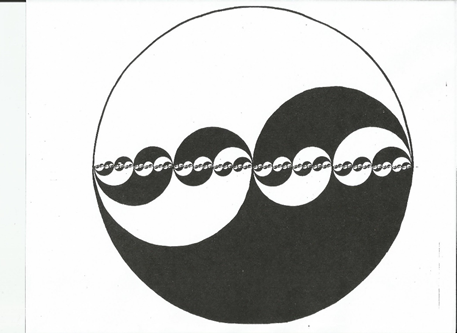This image illustrates binary notation on the unit interval. To find the binary expansion of a real number, locate the number on the horizonal line, then note, for each wave, whether it is above or below the line. For instance, for the point at 2/3, the ½ wave is above the line; the ¼ wave is below the line; the 1/8 wave is above the line; and so on, corresponding to the binary expansion  2/3  =  .10101010… Conversely, with this image one could find the point, given the expansion.

Consider the expansion ½ =  .10000  =  .01111…  This is a form of Zeno’s Paradox: ½  = ¼ + 1/8 + 1/16 + 1/32 + … This double-expansion is at the center of the Octaves image. Just left of the ½ point, the biggest wave is below the line, and all the others are above; and just right of the ½ point, it is the other way around. At every dyadic point (i.e. equal to k/2^n, for some integers k and n), infinitely many bits flip.

I say that Zeno’s Paradox explains Cantor’s Paradox. Any enumeration of the continuum has an antidiagonal with a bit equal to its own negation. The usual reading of that buzzing bit is that the continuum is not denumerable, and there must be infinities beyond infinity. Was more ever made from less? I say that Cantorian bit-flip detects Zenonian bit-flip at a dyadic; so the antidiagonal is a dyadic, the continuum is countable (as the Lowenheim-Skolem theorem allows) and there is only one infinity, and it is paradoxical, as Zeno demands.

A subtler interpretation yields transfinitely simpler mathematics. That is called elegance; sign of a correct theory. But questions remain. Why must infinitely many bits flip at a dyadic? Is it possible to denote the continuum so that at most only one bit flips at any point?

Yes, it is possible! Consider this image; “Odd Dyadic Waves”: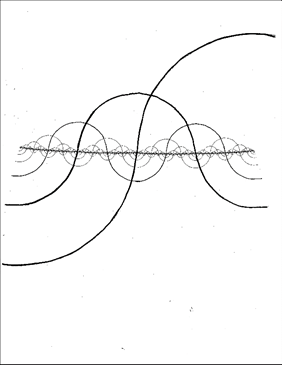The 1/2 wave passes through the line at 1/2; the 1/4 wave passes through the line at 1/4 and 3/4; the 1/8 wave passes through the line at 1/8, 3/8, 5/8, and 7/8; and the 1/2^k wave passes through the line at n/2^k, where n is an odd number. Therefore the name “odd dyadic”, and therefore at any point, at most one bit flips.

Just as with Octaves, this image illustrates a system of binary expansions for any real number on the unit interval. For instance, at the 2/3 point, the 1/2 wave is above the line, as are the 1/4, 1/8, 1/16, … waves; yielding the odd-dyadic expansion 2/3 = .11111…  For the 1/3 point we get the odd-dyadic expansion 1/3 = .01111… The 1/2 point has two odd-dyadic expansions: ½ = .00000… = .10000…

Odd-dyadic notation extends to the integers. Here is a list of the integers from -15 to +15:

- 1000.

- 1001.

- 1011.

- 1010.

- 1110.

- 1111.

- 1101.

- 1100.

- 0100.

- 0101.

- 0111.

- 0110.

- 0010.

- 0011.

- 0001.

- 0000.       =       + 0000.

+ 0001.

+ 0011.

+ 0010.

+ 0110.

+ 0111.

+ 0101.

+ 0100.

+ 1100.

+ 1101.

+ 1111.

+ 1110.

+ 1010.

+ 1011.

+ 1001.

+ 1000.

Only one place changes between successive integers. The +/- sign is a sort of infinity-place for this dyadic-binary notation, which flips at zero, where positive meets negative.

You can transform between binary and odd dyadic notation:

. a1, a2, a3, a4, …  in binary is, in odd dyadic:

. a1,   a1+a2,   a2+a3,  a3+a4, …

. a1, a2, a3, a4, …  in odd dyadic is, in binary:

. a1,   a1+a2,   a1+a2+a3,   a1+a2+a3+a4, …

where + is addition modulo two.

Addition mod 2 is the same as subtraction mod 2 because x+x=0 mod 2 for all x. The binary to odd-dyadic map is the difference between successive places, and the odd-dyadic to binary map is summation of places. Therefore I call odd dyadic the “place difference of binary” and binary the “place summation of odd dyadic”.

Here are more images of how binary and odd-dyadic notation divide up the line.

Horizon: